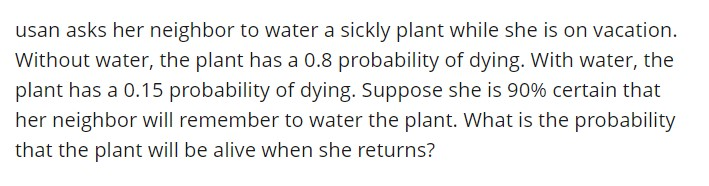# usan asks her neighbor to water a sickly plant while she is on vacation.Without water, the plant has a 0.8 probability of dying. With water, theplant has a 0.15 probability of dying. Suppose she is 90% certain thather neighbor will remember to water the plant. What is the probabilitythat the plant will be alive when she returns?

Question
27 views

Hello. Can I get help on how to calculate this question? If there is also a way to use the TI-83 graphing calculator, it would be helpful! Thank You!help_outlineImage Transcriptioncloseusan asks her neighbor to water a sickly plant while she is on vacation. Without water, the plant has a 0.8 probability of dying. With water, the plant has a 0.15 probability of dying. Suppose she is 90% certain that her neighbor will remember to water the plant. What is the probability that the plant will be alive when she returns? fullscreen
check_circle

star
star
star
star
star
1 Rating
Step 1

Conditional probability of two events:

The conditional probability is the probability of one event given that the other event has already occurred.

Consider two events A and B, the conditional probability of the event A given that B has occurred is obtained as follows:

Step 2

Obtain the probability that the plant will be alive when Usan arrives:

The probability that neighbour remember to water the plant is P(Watered) = 0.9.

The probability that neighbour forgets to water the plant is P(Not watered) = 0.1.

The probability that the plant dies without water is P(plant died | N...

### Want to see the full answer?

See Solution

#### Want to see this answer and more?

Solutions are written by subject experts who are available 24/7. Questions are typically answered within 1 hour.*

See Solution
*Response times may vary by subject and question.
Tagged in

### Statistics